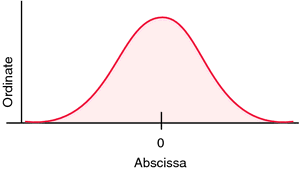# abscissa

(redirected from abscissae)
Also found in: Dictionary, Thesaurus, Encyclopedia.
Related to abscissae: ordinate

## abscissa

[ab-sis´ah]
the horizontal line in a graph along which are plotted the units of one of the variables considered in the study, as time in a time-temperature study. The other line is called the ordinate.Axes of graph showing normal distribution curve.

## ab·scis·sa

(ab-sis'ă),
In a plane cartesian coordinate system, the horizontal axis (x). Compare: ordinate.
[L. ab-scindo, pp. -scissus, to cut away from]

## ab·scis·sa

(ab-sis'ă)
In a plane cartesian coordinate system, the horizontal axis (x).
[L. ab-scindo, pp. -scissus, to cut away from]
Mentioned in ?
References in periodicals archive ?
6) without median tooth at apex; length relation between abscissae of radial vein of fore wing (Fig.
Under some mild assumptions on the coefficients [a.sub.1](x, y), [a.sub.2](x, y), [a.sub.3](x, y) (defining L) and on the mesh refinement strategy it can be shown that, if the collocation abscissae can be chosen to avoid a singular matrix W, then ||[W.sup.-1]|| will be uniformly bounded as h [right arrow] 0, where h = max([D.sub.1], [D.sub.2]).
By a generalization of the above expression for HBW, it can be shown that in a family of visual pigment spectra satisfying the aforementioned assumptions, any two spectra are related such that at equal ordinates, the abscissae vary in proportion to [v.sub.max], i.e., v/[v.sub.max] = V[prime]/[v[prime].sub.max].
If the abscissae [x.sub.i,j] were not subjected to any variation, we would get only a nonfinear amplitude variation of the waveform over time.(13) Since both the abscissae [x.sub.i,j] and the ordinates [y.sub.i,j] of the segment endpoints vary, the polygonal waveform varies both in shape and in duration, leading to amplitude, timbre, and frequency variations of the sound.
The abscissae show time which progresses from right to left (1 cm = 30 secs), while the ordinates indicate both temperature (1 cm = 25 [degrees] C) and power (1 cm = 200 kW).

Site: Follow: Share:
Open / Close